# DRNN Robust DTC for Induction Motor Drive Systems Using FSTPI

DRNN Robust DTC for Induction Motor Drive Systems Using FSTPI
Department of Electrical and Electronics Engineering, University of Eswatini, Kwaluseni M201, Eswatini

Energy and Renewable Energy Department, Faculty of Engineering and Technology, Egyptian Chinese University, Cairo 11321, Egypt

Corresponding Author Email:
zebra.lucky@yahoo.com
Page:
539-547
|
DOI:
https://doi.org/10.18280/jesa.540403
12 October 2020
|
Accepted:
20 July 2021
|
Published:
31 August 2021
| Citation

OPEN ACCESS

Abstract:

In this paper, a self-tuning PI speed controller based on diagonal recurrent neural network is (DRNN) investigated and simulated to increase the robustness of the direct torque control (DTC) scheme for three-phase low-power IM drive system using a Four Switch Three-Phase Inverter (FSTPI). The drive is subjected to different system inputs and disturbances, step changes in speed under different load conditions, abrupt loading at high speed and speed reversal. Furthermore, the robustness of the controller is evaluated by varying motor parameter, stator resistance and moment of inertia. A comparison of classical and self-tuning PI speed controllers was presented to determine the effectiveness of the proposed controller. It is concluded based on simulation results using Matlab/Simulink. that the self-tuning PI speed controller provides the best performance by reacting rapidly and adaptively.

Keywords:

induction motor (IM), direct torque control (DTC), stator resistance (Rs), moment of inertia (J), diagonal recurrent neural network (DRNN)

1. Introduction

The direct torque control (DTC) technique is the most advanced strategy for three-phase induction motor (IM) drive systems in industrial applications. It was first proposed by Takahashi and Noguchi  and Depenbrock  in the mid of 1980s as a replacement of the popular field-oriented control technique. Today, DTC is one of the world's most advanced AC machine drive technologies. It is characterized by its simple and robust structure yet achieves a very fast dynamic torque response. This technique was successfully implemented to drive three-phase induction machine by performing a decoupled control of electromagnetic torque and stator flux of the motor. The induction machines are popular in industrial applications nowadays because they have a rigid structure which makes them reliable and cheap in terms of maintenance . Ever since the DTC technique was introduced, many research studies to improve the conventional DTC strategy have been proposed. Most of the methods to improve the classical DTC that have been introduced are mainly focused on the variable switching frequency of the inverter and high torque ripples resulting from the electromagnetic torque and stator flux hysteresis comparators . The space vector modulation method as proposed by Wang et al. [5-7] has been used to achieve a constant switching frequency and resulting to reduced electromagnetic torque ripples.

In most applications of the DTC technique, the voltage source inverter (VSI) driving the induction machine is a six-switch three-phase inverter (SSTPI) to achieve a high-performance drive system of the motor . A DTC scheme where the induction machine is driven by a four-switch three-phase inverter (FSTPI) was proposed by Metwally . The FSTPI fed drive system is aimed to develop power converters with reduced number of switches and losses which is economic for controlling the speed of a low power and low-cost induction machine drive system while employing the simple and robust DTC strategy . In a FSTPI drive system, two legs of the IM are controlled by power switching devise and the third leg is connected in the middle point of the dc-link voltage. With only two phases of the IM being controlled in FSTPI drive system, the switching table, sector numbers of stator flux position and three-level electromagnetic torque hysteresis controller are reduced which in turn give a fast-processing time compared to a SSTPI fed drive system.

In DTC scheme with FSTPI fed drive system for IM, the optimal switching table is established with four space vectors of the FSTPI in accordance with four sectors to the stator flux position  which is less compared to the six space vectors and six sectors in SSTPI  fed drive system. The electromagnetic torque control is achieved by using a two-level hysteresis comparator. In most research proposal for both the SSTPI and FSTPI fed drive system for IM, the speed regulation is achieved by using a classical proportional integral (PI) controller to generate the torque command. However, a typical PI controller cannot easily achieve a rapid response and an accurate drive system over a wide range of speed, particularly when there are motor parameters uncertainties and unanticipated load changes .

Adaptive artificial intelligence technology to achieve high-performance control of the VSI-powered IM drive system has been developed to address the drawbacks associated with the PI regulator . Artificial-intelligent techniques include, among others, neural network control, fuzzy logic control, and robust mode control. This paper presents a DTC strategy for the FSTPI-powered induction machine drive system, where the closed-loop speed regulator uses an adaptive diagonal recurrent neural network (DRNN) controller. The strategy implemented is based on the four space vector voltages of FSTPI, simply by deriving an effective voltage vector switching table controlled by two-level hysteresis controllers for stator flux and electromagnetic torque combined with stator flux location in the stationery reference frame on a four-level sector.

A detailed comparative analysis of the designed controller is evaluated, considering five different operating conditions of the IM, between the classical and neural network controllers. Fundamental principles of the outlined approaches are presented, and certain features of the designed algorithm's effectiveness are evaluated and demonstrated. The basic principles of the outlined approaches are presented and some features demonstrating the effectiveness of the designed algorithm are evaluated and verified.

2. DTC with FSTPI Fed IM

The dynamic induction motor model used in this paper is described by the following equations in the synchronous reference frame based on :

$V_{q s}^{e}=R_{s} i_{q s}^{e}+\omega_{s} \phi_{d s}^{e}+\mathrm{p} \phi_{q s}^{e}$    (1)

$V_{d s}^{e}=R_{s} i_{d s}^{e}-\omega_{s} \phi_{q s}^{e}+\mathrm{p} \phi_{d s}^{e}$    (2)

$V_{q r}^{e}=0=R_{r} i_{q r}^{e}+\left(\omega_{s}-\omega_{r}\right) \phi_{d s}^{e}+\mathrm{p} \phi_{q s}^{e}$    (3)

$V_{d r}^{e}=0=R_{r} i_{q r}^{e}-\left(\omega_{s}-\omega_{r}\right) \phi_{d s}^{e}+\mathrm{p} \phi_{q s}^{e}$    (4)

$\phi_{q s}^{e}=L_{s} i_{q s}^{e}+L_{m} i_{q r}^{e}$    (5)

$\phi_{d s}^{e}=L_{s} i_{d s}^{e}+L_{m} i_{d r}^{e}$    (6)

$\phi_{q r}^{e}=L_{r} i_{q r}^{e}+L_{m} i_{q s}^{e}$    (7)

$\phi_{d r}^{e}=L_{r} i_{d r}^{e}+L_{m} i_{d s}^{e}$    (8)

$T_{e}=\frac{3 P L_{m}}{4 L_{r}}\left(\phi_{d r}^{e} i_{q s}^{e}+\phi_{q r}^{e} i_{d s}^{e}\right)$    (9)

$T_{e}-T_{L}=\frac{2}{P}\left(J \frac{d \omega_{r}}{d t}+\mathrm{B} \omega_{r}\right)$    (10)

where, $V_{d s}^{e}, V_{q s}^{e}:$ Stator voltages in d-q co-ordinates. $i_{d s}^{e}, i_{q s}^{e}:$ Stator currents in d-q co-ordinates. $\phi_{d s}^{e}, \phi_{q s}^{e}:$ Stator flux linkages in d-q co-ordinates. $V_{d r}^{e}, V_{q r}^{e}:$ Rotor voltages in d-q co-ordinates. $i_{d r}^{e}, i_{q r}^{e}:$ Rotor currents in d-q co-ordinates. $\phi_{d r}^{e}, \phi_{q r}^{e}:$ Rotor flux linkages in d-q co-ordinates. $T_{e}:$ Electromagnetic torque of the induction motor. $T_{L}:$ Load torque. P: Number of poles of the induction motor. $\omega_{s}:$ Stator angular frequency (rad/sec). $\omega_{r}:$ Rotor electrical speed in angular frequency (rad/sec). $R_{s}, R_{r}:$ Stator and rotor resistance referred to the stator. $L_{s}, L_{r}:$ Stator and rotor inductance referred to the stator. $L_{m}:$ Mutual inductance referred to the stator. J: A moment of inertia of the induction motor. B: Motor friction. p=ddt.

The electromagnetic torque produced by the IM is presented as:

$T_{e}=\frac{3}{2} \frac{P}{L_{s}} \frac{L_{m}}{L_{r}} \overline{\phi_{r}} \times \overline{\phi_{s}}=\frac{3}{2} \frac{P}{L_{s}} \frac{L_{m}}{L_{r}}\left|\overline{\phi_{r}} \| \overline{\phi_{s}}\right| \sin \gamma$    (11)

where, γ is the angle between rotor flux and stator flux vectors and $\left|\overline{\phi_{r}}\right|, \left|\overline{\phi_{s}}\right|$ is the rotor and stator flux magnitudes, stator voltage vector, and stator current vector, respectively. Eq. (11) shows that the torque is depends on the magnitude of stator and rotor flux and the angle between the stator and rotor flux vectors.

The equation of induction motor stator flux is given by :

$\overline{\phi_{s}}=\int\left(\bar{V}_{s}-\overline{l_{s}} R_{s}\right) d t$    (12)

The applied voltage vector therefore defines the change in the stator flux vector. As the voltage vector is applied to the system, the stator flux adjusts to increase the phase angle between the stator and rotor flux vectors. Which will increase the torque output .

## 1.pngFigure 1. Block diagram of DTC with FSTPI

The detailed DTC block diagram shown in Figure 1 is for the FSTPI powered three-phase induction motor systems. The drive system is implemented using MATLAB/Simulink software. The controller consists of four parts mainly speed regulator to generate torque command, commonly performed by PI controller, FSTPI, IM and the DTC block. Synchronous reference frame IM model is used for the design.

In DTC block, the stator flux and torque ($\phi_{s}$ and $T_{e}$) are estimated by means of stator, voltage, and stator resistance to compare with flux and torque values. The flux and torque errors $\left(\Delta \phi_{s}\right.$ and $\left.\Delta T_{e}\right)$ achieved are applied to two-level hysteresis comparators for a decoupled control of torque and flux. In the hysteresis comparators electromagnetic torque and stator flux are kept within a hysteresis band $\left(d \phi_{s}\right.$ and $\left.d T_{e}\right)$, around the reference values of flux and torque $\left(\phi_{s}^{*}\right.$ and $\left.T_{e}^{*}\right)$. The most suitable voltage vectors for the FSTPI are selected from a switching look-up table based on the outputs of the hysteresis controllers $\left(H \phi_{s}\right.$ and $\left.H T_{e}\right)$ in combination with the stator flux location ($\theta_{s}$). The hysteresis controller outputs are obtained by implementation of the block diagrams in Figure 2 and Figure 3 for decoupled control of torque and flux, respectively.

## 2.png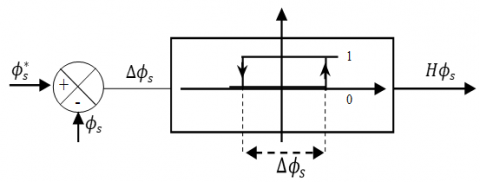Figure 2. Two-level stator flux hysteresis controller

## 3.png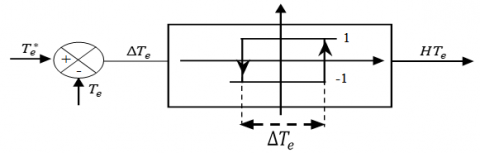Figure 3. Two-level torque hysteresis controller

The space vector of the selected voltage vector to drive the FSTPI is shown in Figure 4 with the switching states and the four sectors.

## 4.png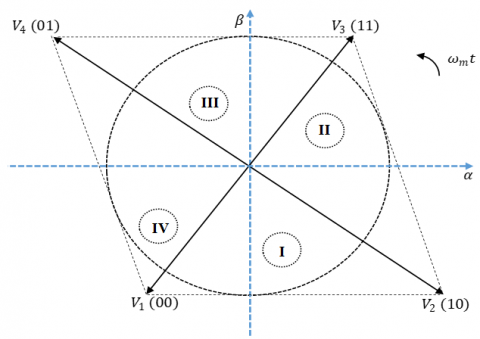Figure 4. Space voltage vectors generated by FSTPI

The main drawback of using a FSTPI in the DTC technique are the high torque and flux ripples produce due to the unbalanced voltages generated by the FSTPI. To reduce the ripples, the DTC switching table implemented is based on the emulation of SSTPI operation by the FSTPI . Figure 5 presents the generation of six balanced voltage vectors by employing the four unbalanced voltages of FSTPI.

## 5.png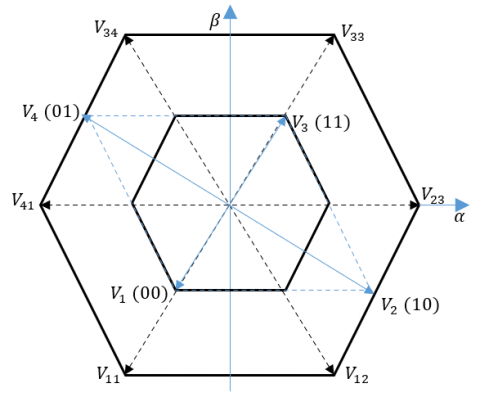Figure 5. Generation of six active voltages from four unbalanced voltages

The FSTPI configuration is given in the Figure 6. As shown, the two phases of the IM are connected to the FSTPI legs with switches and the other phase is connected directly to the midpoint of the dc bus voltage. The IM stator voltage vectors are defined by Eqns. (13) – (18) based on the upper switches which are complementary to the lower switches to avoid a short circuit of the FSTPI.

## 6.png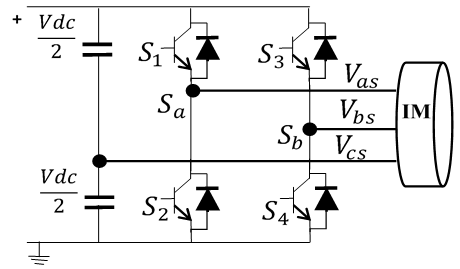Figure 6. FSTPI with three-phase IM connected

The phase-to-zero voltages are a function of the upper switches as follows based on :

$V_{a 0}=\frac{V_{d c}}{2}\left(2 S_{1}-1\right)$    (13)

$V_{b 0}=\frac{V_{d c}}{2}\left(2 S_{3}-1\right)$    (14)

$V_{c 0}=0$    (15)

The phase voltages are a function of the phase-to-zero voltages as follows:

$V_{a s}=\frac{1}{3}\left(2 V_{a o}-V_{b o}\right)$    (16)

$V_{b s}=\frac{1}{3}\left(2 V_{b o}-V_{a o}\right)$    (17)

$V_{c s}=\frac{1}{3}\left(-V_{a o}-V_{b o}\right)$    (18)

3. Design of Classical PI and NN-PI

3.1 Classical PI controller

The PI controller is used to generate the torque command for speed control. The design is based on Figure 7 and Eqns. (19)-(25) and the poles placement method is used to determine the controller gains.

## 7.png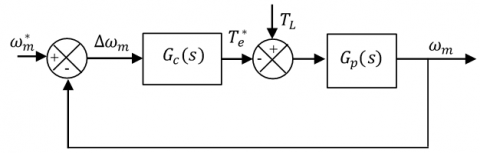Figure 7. Speed control block diagram

The dynamic equation is given as follows:

$\frac{d \omega_{m}}{d t}=-\frac{B}{J} \omega_{m}+\frac{T_{e}}{J}-\frac{1}{J} T_{L}$    (19)

and the transfer function using Laplace transform of the speed loop is given as follows:

$G_{p}(s)=G_{\omega_{m}}(s)=\frac{\omega_{m}(s)}{T_{e}(s)-T_{L}(s)}=\frac{1}{J s+B}$    (20)

The transfer function of the PI controller is defined as follows:

$G_{c}(s)=G_{P I}(s)=K_{p} s+\frac{K_{i}}{s}$    (21)

By considering the load torque $T_{L}$ as a disturbance. The overall transfer function of the speed control in open loop becomes:

$T_{o p}(s)=G_{c}(s) \times G_{\omega_{m}}(s)=\left(K_{p} s+\frac{K_{i}}{s}\right)\left(\frac{1}{J s+B}\right)$    (22)

The transfer function of the speed controller system in closed loop becomes:

$T_{c l}(s)=\frac{G_{c}(s) \times G_{p}(s)}{G_{c}(s) \times G_{p}(s)+1}=\frac{K_{p} s+K_{i}}{s^{2}+\frac{\left(K_{p}+B\right)}{J} s+\frac{K_{i}}{J}}$    (23)

Comparing the denominator of Eq. (23) with the denominator of canonical form of second order system given in Eq. (24):

$T(s)=\frac{1}{s^{2}+2 \xi \omega_{n} s+\omega_{n}^{2}}$    (24)

where, $\omega_{n}$ is the natural sampling frequency and ξ is the damping coefficient. The following can be concluded:

$\left.\begin{array}{c}K_{i}=J \omega_{n}^{2} \\ K_{p}=2 \xi \omega_{n}-B\end{array}\right\}$    (25)

The damping coefficient: $\xi=\frac{1}{\sqrt{2}}$.

3.2 DRNN-PI speed controller

The DTC structure speed controller is a non-linear system that has load inertia disturbances and variations of motor temperature parameters . This makes it hard for the DTC control system to work adequately with the PI speed controller. The paper in hand proposes a diagonal recurrent neural network (DRNN) adaptive speed controller system, which is confirmed by simulation.

There are two parts to the NN-PI speed controller with DRNN algorithm One is the classic PI controller that controls the motor speed in the closed loop. The second part is DRNN that can adjust online the $K_{p}$ and $K_{i}$ parameters to ensure optimum performance. The NN-PI speed controller is schematically structured, as shown in Figure 8. This controller can react rapidly, adaptively and counter-interference .

## 8.png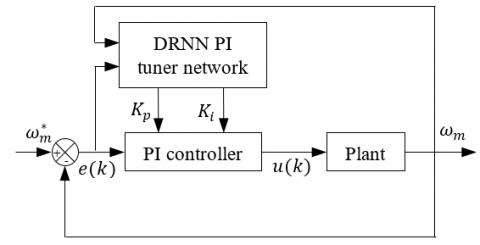Figure 8. Auto-tuning structure DRNN-PI speed controller

If r(k) is given speed reference $\omega_{m}^{*}$ and $y(k)$ is given actual output speed $\omega_{m}$, then the control error is:

$e(k)=r(k)-y(k)$    (26)

The two inputs of the PI controller are:

$x_{1}(k)=e(k)$    (27)

$x_{2}(k)=\sum_{i=1}^{k} e(k) \times T_{s}$    (28)

$T_{s}$ is sampling time. The control algorithm for tuning $K_{p}$ and $K_{i}$ online using DRNN is:

$u(k)=K_{p}(k) x_{1}(k)+K_{i}(k) x_{2}(k)$    (29)

The loss function using sum squared error can be defined as:

$E(k)=\frac{1}{2}(r(k)-y(k))^{2}=\frac{1}{2} e(k)^{2}$    (30)

The adjustment of $K_{p}$ and $K_{i}$ adopts the gradient descent method:

$D K_{p}=-\eta \frac{\partial E}{\partial K_{p}}=-\eta \frac{\partial E}{\partial y} \times \frac{\partial y}{\partial u} \times \frac{\partial u}{\partial K_{p}}=\eta e(k) \frac{\partial y}{\partial u} x_{1}(k)$    (31)

$D K_{i}=-\eta \frac{\partial E}{\partial K_{i}}=-\eta \frac{\partial E}{\partial y} \times \frac{\partial y}{\partial u} \times \frac{\partial u}{\partial K_{i}}=\eta e(k) \frac{\partial y}{\partial u} x_{2}(k)$    (32)

η is the learning rate, $\partial y / \partial u$ is the Jacobian information of controlled object, which can be obtained by using DRNN, which is trained by the dynamic backpropagation (DBP) algorithm .

The DRNN is made of three layers namely, input, hidden, and output layer and the network structure are shown in Figure 9.

## 9.png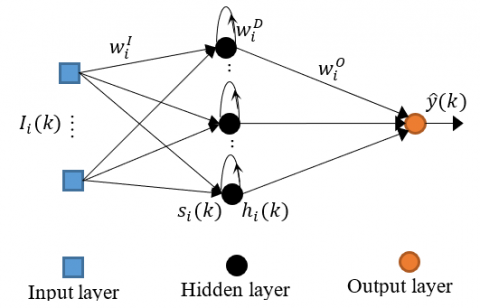Figure 9. Neural network structure

Let $s_{i}(k)(i=1,2, \ldots, h)$ where $h$ is the number of hidden neurons) represent the input sum of the recurrent neuron, $h_{i}(k)(i=1,2, \ldots, h)$ the output of hidden neuron, and $\hat{y}(k)$ the output of the network. Then the output of the ith hidden neuron can be written as:

$h_{i}(k)=f(x)=f\left(s_{i}(k)\right)$    (33)

$s_{i}(k)=w_{i}^{D} h_{i}(k-1)+\sum_{j=1}^{m+1} w_{i, j}^{I} I_{i}(k)$    (34)

where, $w_{i, j}^{I}$ is the weight connecting the $j$ th input to the ith hidden neuron, $w_{i}^{D}$ is the feedback weight of the ith hidden neuron, bias weight is zero, and f(x) is the sigmoid function. The network output can be written as:

$\hat{y}(k)=\sum_{j=1}^{h} w_{i}^{O} h_{i}(k)$    (35)

where, $w_{i}^{O}$ is the output layer weight connecting the jth hidden neuron and the output neuron.

The objective of the network training is to minimize the following error function:

$J(k)=\frac{1}{2}(d(k)-\hat{y}(k))^{2}=\frac{1}{2} e_{I}^{2}(k)$    (36)

d(k) is the desired network output.

Applying the DBP learning algorithm, the learning rules of the weights are obtained as follows:

(1) Output weights:

$w_{i}^{O}(k+1)=w_{i}^{O}(k)-\eta \frac{\partial J(k)}{w_{i}^{O}}=w_{i}^{O}(k)+\eta e_{I}(k) \frac{\partial \hat{y}(k)}{\partial w_{i}^{O}}$    (37)

$\frac{\partial \hat{y}(k)}{\partial w_{i}^{O}}=h_{i}(k-1), i=1,2, \ldots, h$    (38)

(2) Feedback weights:

$w_{i}^{D}(k+1)=w_{i}^{D}(k)-\eta \frac{\partial J(k)}{w_{i}^{D}}=w_{i}^{D}(k)+\eta e_{I}(k) \frac{\partial \hat{y}(k)}{\partial w_{i}^{D}}$    (39)

$\frac{\partial \hat{y}(k)}{\partial w_{i}^{D}}=w_{i}^{O} P_{i}(k-1), i=1,2, \ldots, h$    (40)

$P_{i}(k)=\frac{\partial h_{i}(k)}{\partial w_{i}^{D}} f^{\prime}\left(s_{i}(k)\right)\left(h_{i}(k-1)\right)+w_{i}^{D} P_{i}(k-1)$    (41)

(3) Input weights:

$w_{i, j}^{I}(k+1)=w_{i, j}^{I}(k)-\eta \frac{\partial J(k)}{w_{i, j}^{I}}=w_{i, j}^{I}(k)+\eta e_{I}(k) \frac{\partial \hat{y}(k)}{\partial w_{i, j}^{I}}$    (42)

$\frac{\partial \hat{y}(k)}{\partial w_{i, j}^{I}}=w_{i}^{O} Q_{i, j}(k-1) ; i=1,2, \ldots, h, \quad j=1,2, \ldots, m$    (43)

$Q_{i, j}(k)=\frac{\partial h_{i}(k)}{\partial w_{i, j}^{I}} f^{\prime}\left(s_{i}(k)\right)\left(I_{i}(k)\right)+w_{i}^{D} Q_{i, j}(k-1)$    (44)

The above training algorithm can be used both offline and online. An online weight update detects adjustments in the dynamic phase and therefore leads to adaptive regulation.

The Jacobian information of the controlled object value is:

$\frac{\partial y}{\partial u} \approx \frac{\partial \hat{y}}{\partial u}=\sum_{i} w_{i}^{O} f^{\prime}\left(s_{j}\right) w_{i, j}^{O}$    (45)

4. Results

To evaluate the effectiveness and robustness of the DTC technique with self-tuning PI speed controller based on DRNN, the controller is tested under five different operating conditions of the IM. The results are shown in Figures 10 to 16. The figures are specified: (a) for DTC technique using FSTPI with classical PI speed regulator and (b) for DTC technique using FSTPI with self-tuning PI speed regulator based on DRNN.

Case (1)

In this case the motor speed is increased from 100 rad/sec to 140 rad/sec at t = 0.4 sec. It is decreased back to 100 rad/sec at t = 0.8 sec. The torque is kept constant at 4 N.m. The speed and torque characteristics are shown in Figure 10 and Figure 11, respectively.

Case (2)

In this case the motor speed is kept constant at 138 rad/sec, while the electromagnetic torque is increased from 3 N.m to 6 N.m at t = 0.4 sec, and decreased back to 3 N.m at t = 0.8 sec Figure 12 and Figure 13 shows the characteristic of speed and torque response for this case, respectively.

Case (3)

In this instance the motor speed is reversed from 100 rad/sec to -100 rad/sec at t = 0.2 sec while the motor load torque is kept constant at 2 N.m. The speed response is demonstrated in Figure 14.

Case (4)

The motor stator resistance is varied at 50%, 100%, and 150% of actual value in this case. The motor speed is kept constant at 120 rad/sec and the motor load torque is also kept constant at 5 N.m. Speed response is presented in Figure 15 for this case and the targeted speed is achieved.

Case (5)

The moment of inertia for the motor is varied at 50%, 100%, and 150% of actual value in this case. The motor speed is kept constant at 120 rad/sec and the motor load torque is also kept constant at 5 N.m. For this case, the speed response is shown in Figure 16.

## 10.pngFigure 10. Motor speed response (a) with classical PI (b) with NN-PI for step changes in motor speed.

## 11.pngFigure 11. Electromagnetic torque response (a) with classical PI (b) with NN-PI for step changes in motor speed.

## 12.png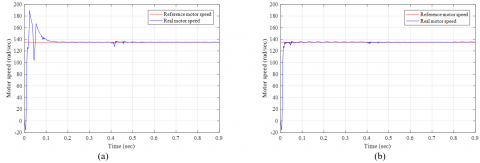Figure 12. Motor speed response (a) with classical PI (b) with NN-PI for step changes in motor load torque.

## 13.png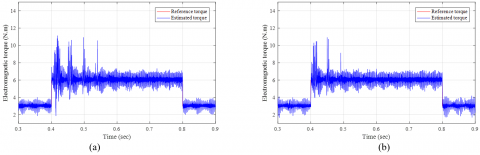Figure 13. Electromagnetic torque response (a) with classical PI (b) with NN-PI for step changes in motor load torque.

## 14.png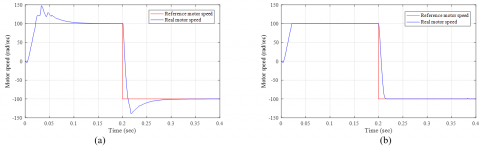Figure 14. Motor speed response (a) with classical PI (b) with NN-PI for motor speed reversal.

## 15.png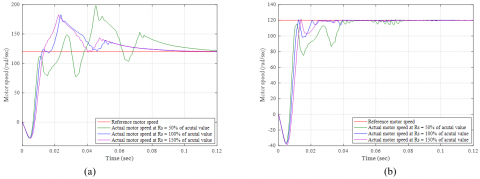Figure 15. Motor speed response (a) with classical PI (b) with NN-PI for stator resistance variation.

## 16.png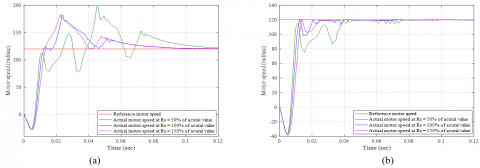Figure 16. Motor speed response (a) with classical PI (b) with NN-PI for moment of inertia variation

The effectiveness and validity of the self-tuning PI speed controller based on DRNN is presented in the results shown by the figures from Figure 10 to Figure 16. Another observation, there is less overshoot in DRNN based PI speed controller, hence it reaches steady state quicker compared to classical PI controller as shown by Figures 10, 12, 14, 15 and 16. Furthermore, Figures 11 and 13 shows less torque ripple in DRNN based PI controller compared to classical PI controller. The robustness of the DRNN-PI speed controller is demonstrated by Figure 15 and Figure 16. In these cases, the stator resistance and moment of inertia parameters for the motor are varied between 50%, 100% and 150%, and as demonstrated in these figures, the controller based in DRNN achieved the targeted speed with better performance in comparison with the classical PI controller.

5. Conclusions

A development of a robust DTC controller for three-phase induction motor using a FSTPI with self-tuning PI controller based on DRNN for speed regulation has been presented. To minimize torque ripples compared with the classical DTC technique for FSTPI, the DTC look-up table implemented is based on the emulation of SSTPI operation by the FSTPI. The validity of the proposed motor drive control system is designed and simulated using Matlab/Simulink model. Comparative results between using classical PI and self-tuning PI speed regulator based on DRNN, shows that the robustness of the controller is enhanced with self-tuning PI controller. The DTC scheme with self-tuning PI speed controller based on DRNN provides a better response with reduced overshoot and faster settling time over using a classical PI controller.

Nomenclature
 DTC Direct Torque Control PI Proportional Integral IM Induction Motor DRNN Diagonal Recurrent Neural Network FSTPI Four Switch Three-Phase Inverter SSTPI Six Switch Three-Phase Inverter NN-PI Neural Network Proportional Integral VSI Voltage Source Inverter
Appendix

The parameters of the induction motor are presented in Table 1.

Table 1. IM Parameters

 Motor Parameter Value Power 1.2 kW Voltage, (V) 240 V Frequency (f) 50 Hz Stator resistance ($R_{s}$) 11.7 Ω Rotor resistance ($R_{r}$) 10.4 Ω Stator self-inductance ($L_{s}$) 579 mH Rotor self-inductance ($L_{r}$) 579 mH Mutual inductance ($L_{m}$) 557 mH Moment of inertia (J) $0.0165 \mathrm{~kg} \mathrm{~m}^{2}$ Motor friction (B) 0.0089 N.m/rad/sec Number of poles (P) 4
References

 Takahashi, I., Noguchi, T. (1986). A new quick-response and high-efficiency control strategy of an induction motor. IEEE Transactions on Industry Applications, IA-22(5): 820-827: https://doi.org/10.1109/TIA.1986.4504799

 Depenbrock, M. (1988). Direct self-control (DSC) of inverter-fed induction machine. IEEE Transactions on Power Electronics, 3(4): 420-429: https://doi.org/10.1109/63.17963

 Dube, L., Bayoumi, E. (2020). Robust DTC against parameter variation for three phase induction motor drive systems. 2020 International Conference on Electrical, Communication, and Computer Engineering (ICECCE). https://doi.org/10.1109/ICECCE49384.2020.9179205

 Charmi, S., Badsi, B.E., Yangui, A. (2018). Direct torque control strategy based on the emulation of six-switch inverter operation by a four-switch inverter using an adaptive fuzzy controller. Advances in Science, Technology and Engineering Systems Journal, 3(2): 346-356: https://doi.org/0.25046/aj030237

 Wang, F., Zhang, Z., Mei, X., Rodriguez, J., Kennel, R. (2018). Advanced control strategies of induction machine: field oriented control, direct torque control and model predictive control. Energies, 11(1): 120: https://doi.org/10.3390/en11010120

 Casadei, D., Serra, G., Tani, K. (2000). Implementation of a direct control algorithm for induction motors based on discrete space vector modulation. IEEE Transactions on Power Electronics, 15(4): 769-777: https://doi.org/10.1109/63.849048

 Habetler, T.G., Profumo, F., Pastorelli, M., Tolbert, L.M. (1991). Direct torque control of induction machines using space vector modulation. Conference Record of the 1991 IEEE Industry Applications Society Annual Meeting, 1: 428-436: https://doi.org/10.1109/IAS.1991.178191

 Metwally, M.K. (2014). Direct torque control of four switch three phase inverter fed induction motor sensorless speed drive. International Journal of Power Electronics and Drive System. 5(2): 153-165.

 Dzung, P.Q., Phuong, L.M., Vinh, P.Q., Hoang, N.M., Bac, N.X., (2007). A New Switching Technique for Direct Torque Control of Induction Motor using Four-Switch Three-Phase Inverter. 2007 7th International Conference on Power Electronics and Drive Systems, 1331-1336. https://doi.org/10.1109/PEDS.2007.4487877

 Toufouti, R., Meziane, S., Benalla, H., Algeria, C. (2006). Direct torque control for induction motor using fuzzy logic. Journal of Automatic Control and System Engineering, 6(2): 19-26.

 Bayoumi, H.E. (2014). Stator resistance estimator for direct torque control of permanent magnet synchronous motor drive systems using multi-resolution analysis wavelet. International Journal of Industrial Electronics and Drives, 1(3): 191-201: https://doi.org/10.1504/IJIED.2014.064092

 Bayoumi, H.E. (2014). Multi-resolution analysis wavelet PI stator resistance estimator for direct torque induction motor drive. WSEAS Transactions on Circuits and Systems, 12(7): 211-220.

 Lee, B.S., Krishna, R. (1998). Adaptive stator resistance compensator for high performance direct torque controlled induction motor drives. Conference Record of 1998 IEEE Industry Applications Conference. Thirty-Third IAS Annual Meeting (Cat. No.98CH36242), 1: 423-430: https://doi.org/10.1109/IAS.1998.732337

 Chen, C. (2015). Self-tuning PID control of induction motor speed control system based on diagonal recurrent neural network. International Journal of Control and Automation, 8(10): 321-334: http://dx.doi.org/10.14257/ijca.2015.8.10.30

 Ku, C.C., Lee, K.Y. (1995). Diagonal recurrent neural networks for dynamic stems control. IEEE Transactions on Neural Networks, 6(1): 144-156. https://doi.org/10.1109/72.363441